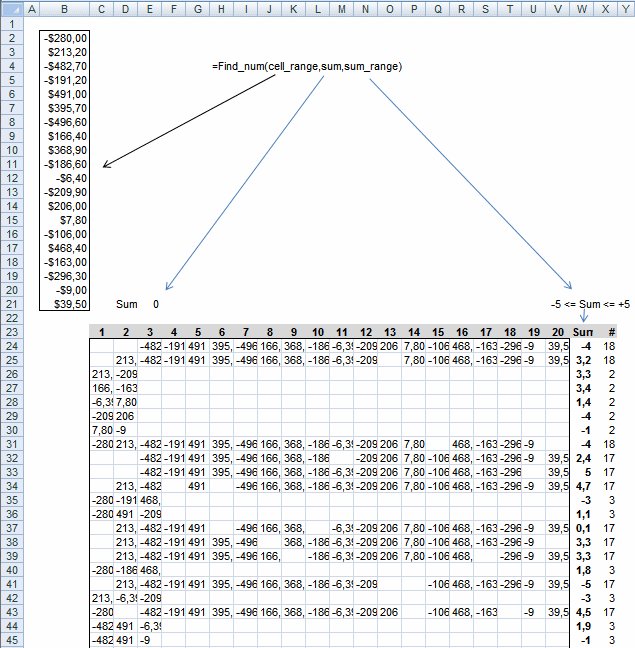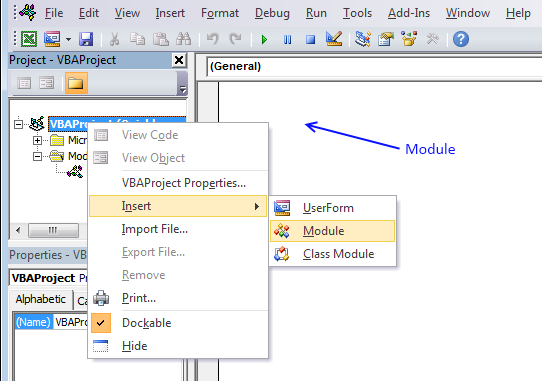Author: Oscar Cronquist Article last updated on January 11, 2019Question:I would like to reduce the number of open items by identifying positive and negative amounts that net to zero and clear those items

Criteria:

• Minimze the number of open items
• include as many transactions as possible
• net as close to zero as possible

This article describes a UDF (custom function) that finds positive and negative numbers that net to approximately zero. The UDF lets you specify the range to use around 0 (zero).

I created/modified an UDF to solve his question: Find positive and negative amounts that net to zero in excel

Array formula in cell range c23:V55:

=FIND_num(B2:B21, E21, 5)

To enter an array formula, type the formula in a cell then press and hold CTRL + SHIFT simultaneously, now press Enter once. Release all keys.

The formula bar now shows the formula with a beginning and ending curly bracket telling you that you entered the formula successfully. Don't enter the curly brackets yourself.

The udf processes these 20 numbers in around 30 seconds. There are 18 numbers found in row 24 and also in row 25 and 31. Formula in column W sums values in each particular row. Formula in column X counts values.1. Press Alt-F11 to open visual basic editor
2. Press with left mouse button on Module on the Insert menu
3. Copy and paste the belowuser defined function
4. Exit visual basic editor

VBA code

```Function Find_num(rng As Range, cell As Range, num_range As Single)
Dim com() As Single, c As Single, i As Single
Dim s As Single, d As Single, u As Single, v As Single
Dim arr() As String, r As Single, p As Single, t As Single
Dim sum_cells As Single, j As Single, k As Single, l As Single
sum_cells = Application.WorksheetFunction.Sum(rng)
c = rng.Rows.Count
ReDim arr(1 To 10000, 1 To c)
r = 1
For i = 0 To Int(c / 2)
If i <> Int(c / 2) - 1 Then
t = WorksheetFunction.Combin(c, i + 1)
Else
t = WorksheetFunction.Combin(c, i + 1) / 2
End If
ReDim com(i)
For j = 0 To i
com(j) = j + 1
Next
k = i
For s = 1 To t
If com(k) > c And i > 0 Then
p = 0
Do Until com(k) <= c - p
com(k - 1) = com(k - 1) + 1
k = k - 1
p = p + 1
Loop
Do Until k >= i
k = k + 1
com(k) = com(k - 1) + 1
Loop
End If
d = 0
For j = 0 To i
d = d + rng(com(j))
Next j
If d <= cell + num_range And d >= cell - num_range Then
For j = 1 To i + 1
arr(r, j) = rng(com(j - 1))
Next j
r = r + 1
End If
If sum_cells - d <= cell + num_range And sum_cells - d >= cell - num_range Then
For j = 1 To c
v = 0
For u = 0 To i
If rng(com(u)) = rng(j) Then v = 1
Next u
If v = 0 Then
arr(r, j) = rng(j)
End If
Next j
r = r + 1
End If
com(k) = com(k) + 1
Next s
Next i
Find_num = arr()
End Function
```

### Get the Excel fileFind-amounts-netting-to-zero-.xls